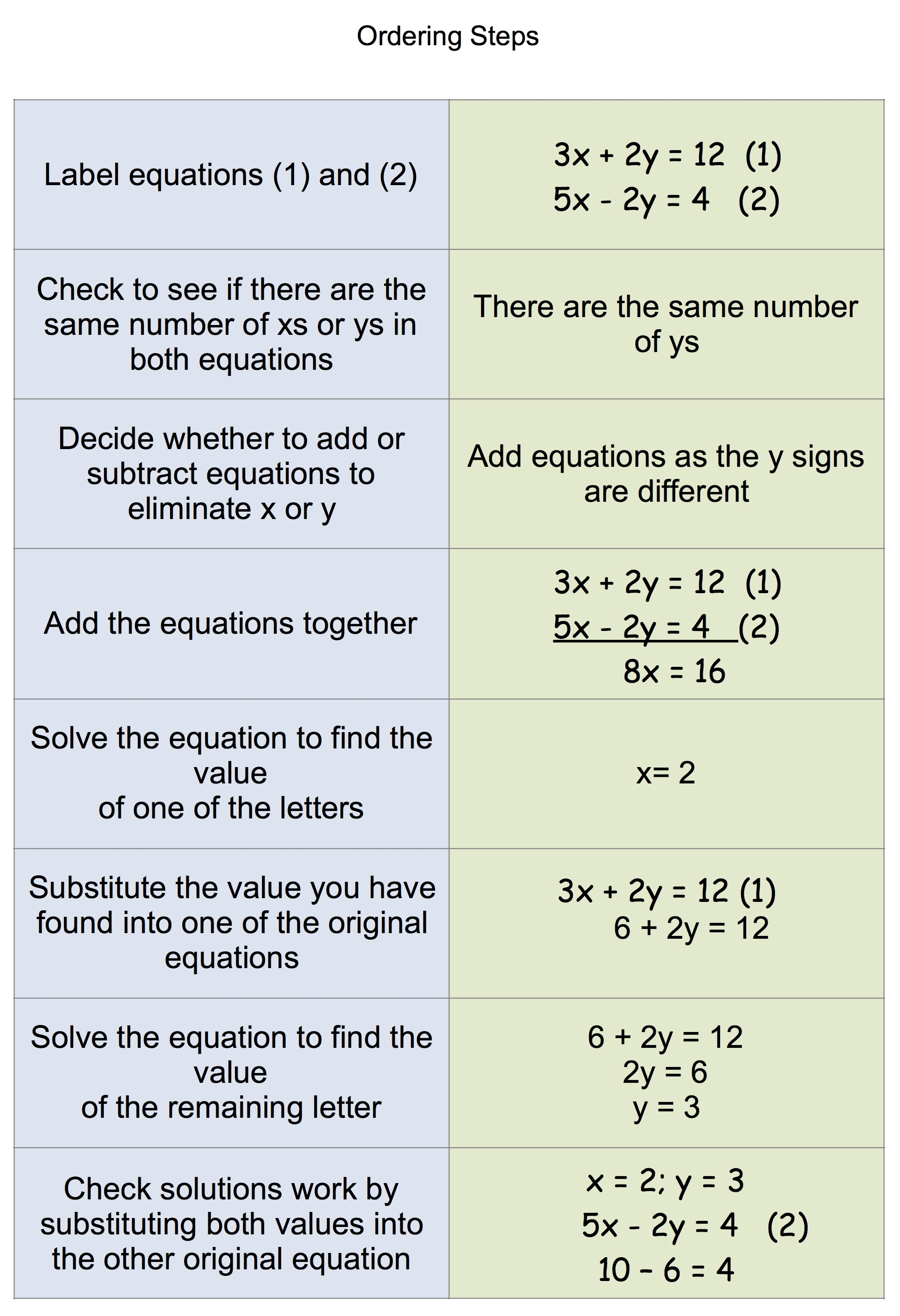This is the course page for your Level 3 Maths Algebra workshop. You can follow the links below to access the resources you need for this session.

### Session PowerPoint

L3 Algebra online presentation Apr18

### Carousel of activities (R1 – 6)

R1 – 6 below are all resources that you can print off, cut up (where relevant) and use to practice aspects of algebra.
R1 Algebra Racetrack

### Simultaneous equations (R8 – 9)

R8 – Have a look at the simultaneous equations in the image below and be prepared to talk about the ordering steps.R9 Problems involving simultaneous equations

Solve the following problems using simultaneous equations

In each case represent each unknown with a letter. Form two separate equations and then follow the usual steps to solve them simultaneously.

1. In an election Mrs White was elected with a majority of 346 over Mr Brown. The total number of votes cast was 1418. How many votes did each candidate receive?
2. On a package holiday, two adults and one child can go for £1190. Similarly, the fare for one adult and three children is £1320. How much does it cost for an adult and a child?
3. The staff room coffee machine takes 20p or 50p coins. When emptied, it was found to contain 36 coins totalling £10.50 in value. How many of each sort of coin did the machine contain?

You can find the answers by clicking the torch icon below.